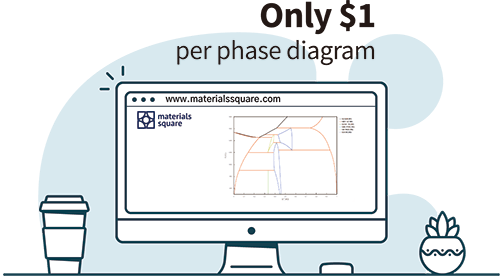Calphad - Materials Square
Features

# On-Demand Databases on Cloud

### Get phase diagram on web

You can produce List-equilibrium, Binary, Ternary, Multi, Function and User-defined phase diagram easily on web.

### Pay only per phase diagram

Don't worry about database and software fees anymore. Pay only for the number of phase diagram you get.

# What kind of phase diagram do you need?

Calphad is a method of CALculating PHAse diagrams. MatSQ Calphad provides six types of phase diagrams.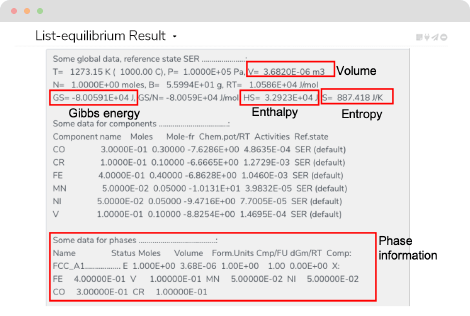### List-Equilibrium

You can calculate the information of each phase on a temperature condition.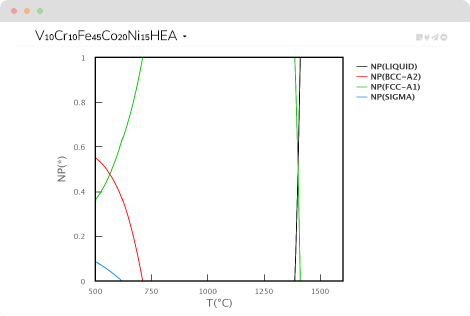### Multi component phase diagram

You can calculate each phase information of the microstructure of alloy with various elements added according to the temperature.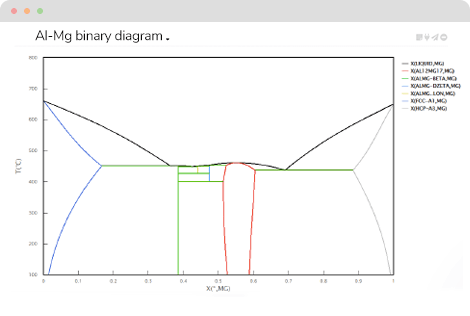### Binary phase diagram

You can calculate the phase diagram between two elements.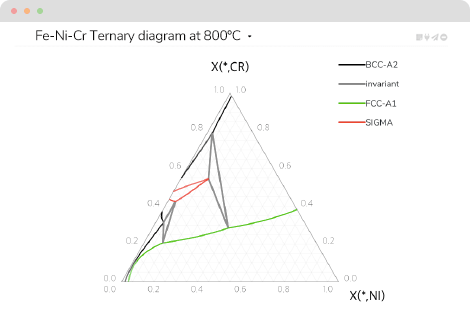### Ternary phase diagram

You can calculate the phase diagram between three elements.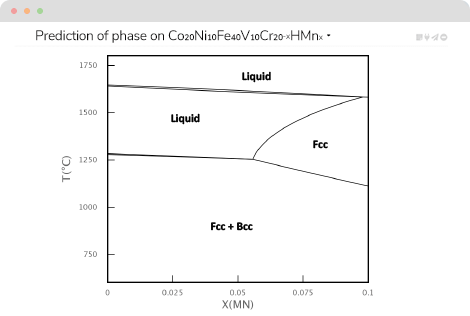### User-defined diagram

You can calculate the phase information of alloy according to the custom settings.

To get user-defined phase diagram ⇢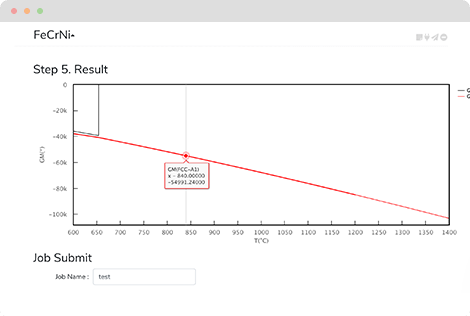### Function diagram

You can calculate energy function (G, H, S, activity, etc) according to the temperature or composition.

Type of functions ⇢

Pricing

# Pay Only per Phase Diagram

You can get a phase diagram at a price of \$0.1 up to \$ 2 which depends on the database.

See more ⇢